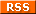olivier godechot# Some useful functions for R

I build from time some small functions and make them available.

## Lags and forwards

# Simplelag gets a variable value from a given line above. Forward gets the value from a given line below. It's inspired from the lag and forward function in SAS.

#mylag: number of lags

#myforward: number of forward

#by: enables to do a lag or of forward by a grouping variable

#outside: default value when lags or forwards are not available

`simplelag <-function(x,by=NULL,mylag=1,outside=NA){  myend<-length(x)- mylag  if (!is.null(by)) {    lby<-c(replicate(mylag,""),as.character(by[1:myend]))    y0<-c(replicate(mylag,outside),x[1:myend])    y<-ifelse(as.character(by)==lby,y0,outside)      }  else {    y<-c(replicate(mylag,outside),x[1:myend])  }}forward<-function(x,by=NULL,myforward=1,outside=NA){  mystart<-myforward+1  if (!is.null(by)) {    fby<-c(as.character(by[mystart:length(x)]),replicate(myforward,""))    y0<-c(x[mystart:length(x)],replicate(myforward,outside))    y<-ifelse(as.character(by)==fby,y0,outside)  }  else {    y<-c(x[mystart:length(x)],replicate(myforward,outside))  }}`

#Example

`#Value of d\$x one row aboved\$lag_x<-simplelag(d\$x) `
`#Value of d\$x three rows above, 0 in the two first rowsd\$lag3_x<-simplelag(d\$x,mylag=3, outside=0)`
`#Value of d\$x two rows belowd\$fwd2_x<-forward(d\$x,myforward=2) #Value of d\$x one row above if in the same group, 0 otherwised\$lag_x<-simplelag(d\$x,by=d\$group,outside=0) `

## Retain and obtain

Retain is the equivalent of the retain instruction in SAS. Obtain is the opposite of Retain.

`retain<-function(x,event,outside=NA){  indices <- c(1,which(event==TRUE), length(x)+1)  values <- c(outside,x[event %in% TRUE])  y<- rep(values, diff(indices)) }obtain<-function(x,event,outside=NA){  indices <- c(0,which(event==TRUE), length(x))  values <- c(x[event %in% TRUE],outside)  y<- rep(values, diff(indices)) } `

#Example

`#Retain in the following rows the last x where event =1d\$last_x<-retain(d\$x,d\$event==1)`
`#Obtain in the first rows the first x where event =2d\$first_x<-obtain(d\$x,d\$event==2)`

## Winsoring

`winsor<-function(x,tmax=0.99,tmin=0.01){  y<-pmin(x,quantile(x,probs=tmax,na.rm=TRUE))  y<-pmax(y,quantile(y,probs=tmin,na.rm=TRUE))}`

## egen : Statistics by group

# DEVALUATED. I discovered one could do exactly the same things with the function ave which is in the R default package.

`d\$sd_x<-ave(d\$x,d\$category,FUN=sd)`

# Egen produces statistics of a variable by group, such as mean, median, standard deviation, etc. It is the equivalent of Stata's egen function.

`egen<-function (x,y,myfun=mean){  data_in<-data.frame(x,y)  data_out<-within(data_in,{z = ave(x,y,FUN=myfun,na.rm=TRUE)} )  return(data_out\$z)}`

#Example

`#Mean of x by categoryd\$mean_x<-egen(d\$x,d\$category)`
`#Standard deviation of x by categoryd\$sd_x<-egen(d\$x,d\$category,myfun=sd)`
`#Median of x by categoryd\$median_x<-egen(d\$x,d\$category,myfun=median)`

## Triming

`trim <- function (x) gsub("^\\s+|\\s+\$", "", x)`

## Reversing a string

`strReverse <- function(x) sapply(lapply(strsplit(x, NULL), rev), paste, collapse="") `

## Clustering

# clx and mclx enable to robust-cluster standard errors in a regressions. Those functions are not mine. clx clusters according to one variable, mclx according to two variables.

`clx <-  function(fm, dfcw, cluster){    library(sandwich)    library(lmtest)    M <- length(unique(cluster))    N <- length(cluster)    dfc <- (M/(M-1))*((N-1)/(N-fm\$rank))    u <- apply(estfun(fm),2,               function(x) tapply(x, cluster, sum))    vcovCL <- dfc*sandwich(fm, meat=crossprod(u)/N)*dfcw    coeftest(fm, vcovCL) }mclx <-  function(fm, dfcw, cluster1, cluster2){    library(sandwich)    library(lmtest)    cluster12 = paste(cluster1,cluster2, sep="")    M1 <- length(unique(cluster1))    M2 <- length(unique(cluster2))    M12 <- length(unique(cluster12))    N <- length(cluster1)    K <- fm\$rank    dfc1 <- (M1/(M1-1))*((N-1)/(N-K))    dfc2 <- (M2/(M2-1))*((N-1)/(N-K))    dfc12 <- (M12/(M12-1))*((N-1)/(N-K))    u1 <- apply(estfun(fm), 2,                function(x) tapply(x, cluster1, sum))    u2 <- apply(estfun(fm), 2,                function(x) tapply(x, cluster2, sum))    u12 <- apply(estfun(fm), 2,                 function(x) tapply(x, cluster12, sum))    vc1 <- dfc1*sandwich(fm, meat=crossprod(u1)/N )    vc2 <- dfc2*sandwich(fm, meat=crossprod(u2)/N )    vc12 <- dfc12*sandwich(fm, meat=crossprod(u12)/N)    vcovMCL <- (vc1 + vc2 - vc12)*dfcw    coeftest(fm, vcovMCL)}`
` `
` `

Français | English

NewsOgO: plus ici|more here

[Presse] Germain, Sabine. 2020. "ENTREPRISES Baisse de rémunération : les petits arrangements des grands patrons". Alternatives économiques. 11/06/2020" Germain, Sabine. ...: plus ici|more here

[Publications] Godechot, Olivier et al., , The Great Separation: Top Earner Segregation at Work in High-Income Countries: plus ici|more here

[Publications] Safi, Mirna, Philippe Coulangeon, Olivier Godechot, Emanuele Ferragina, Emily Helmeid, Stefan Pauly, Ettore Recchi, Nicolas Sauger et Jen Schradie, La: plus ici|more here

[Publications] Safi Mirna, Philippe Coulangeon, Olivier Godechot, Emanuele Ferragina, Emily Helmeid, Stefan Pauly, Ettore Recchi, Nicolas Sauger and Jen Schradie, When: plus ici|more here

Tweets (rarely/rarement): @OlivierGodechot